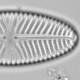## Falling for ggplot2

I spent a long time resisting the lure of ggplot2. I was proficient with the plotting functions in base graphics; why did I need to learn an entirely new graphics system? Yes, getting up colour ramps could be a real pain, but I was in complete control.

Recently I’ve had to learn how to use ggplot2. Students were asking me to help them fix their code (often copied off Stack Overflow), so I needed at least some understanding. I read the book – eller hente ebok frå Universitetsbiblioteket. Its very readable. Now I see why ggplot2 is so popular and have started to use it whenever possible.

Today I wanted to make a screeplot for a principal component analysis in ggplot. So I wrote my own function based on screeplot in the vegan package.

```ggscreeplot <-
function (x, bstick = FALSE, npcs = min(10, if (is.null(x\$CCA) || x\$CCA\$rank == 0) x\$CA\$rank else x\$CCA\$rank),
xlab = "Component", ylab = "Inertia", title = "",  ...) {
if (is.null(x\$CCA) || x\$CCA\$rank == 0)
eig.vals <- x\$CA\$eig
else eig.vals <- x\$CCA\$eig
ncomps <- length(eig.vals)   if (npcs > ncomps)
npcs <- ncomps
comps <- seq(len = npcs)

df <- data.frame(Inertia = eig.vals[comps], names = reorder(factor(names(eig.vals[comps])), comps))
ymax <- df\$Inertia   if (bstick && !is.null(x\$CCA) && x\$CCA\$rank > 0) {
warning("'bstick' unavailable for constrained ordination")
bstick <- FALSE
} else {
df <- cbind(df, bstick = bstick(x)[comps])
ymax <-max(ymax, df\$bstick)
}
g <- ggplot(df, aes(x = names)) +
geom_bar(aes(y = Inertia), stat = "identity", fill = "grey50") +
labs(x = xlab, y = ylab, title = title) +
scale_y_continuous(limits = c(0, ymax * 1.04), expand = c(0, 0))

if (bstick) {
g <- g + geom_point(aes(y = bstick), colour = "red")
g <- g + geom_line(aes(y = bstick, group =1), colour = "red")
}
g
}
```

Here it is in action

```library(rioja)
data(RLGH)#Round Loch of Glenhead diatom stratigraphy
ggscreeplot(rda(sqrt(RLGH\$spec)), bstick = TRUE)
```Screeplot of a PCA of the Round Loch of Glenhead diatom stratigraphy. Note that axis one is much longer than the other axes – it has almost half the total variance.

I’ll be using this code when I show ordinations of the Lake Żabińskie chironomid reconstruction. Spoiler – they don’t look much like this.